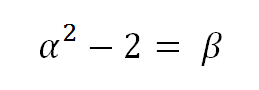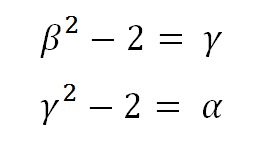## Tuesday, September 11, 2012

### Shuffling the Roots

I've been writing about the theory of equations and how group theory relates to the question of the solvability of polynomials. I'm trying to do it without falling back on the specialized vocabulary of group theory because I want to see what's actually happening. I took a course many years ago where we proved that you can't solve a fifth degree equation, but I never understood the proof. It was too abstract. I want to understand what is actually happening.

There is one enormously important idea that goes through all of this, and it is what I am going to call the idea of being able to shuffle the roots of an equation. The most obvious example is a quadratic equation with two complex roots. There is no mathematical sense in which we can tell those roots apart. They are different numbers, but anything you say about one is equally true of the other.

What is not so obvious is that the same is true, at least in an algebraic sense, about a quadratic equation with two real (but irrational) roots. Even if one root is positive and one is negative, that doesn't distinguish them algebraically. We can flip them with each other and any true statement algebraic statement about either or both of them (e.g. "they add up to 7") remains true before and after flipping them.

The situation gets even more interesting when we go to the third degree equation. Consider the three cube roots of two. It's not surprising that any true algebraic statement involving the two imaginary roots remains true after swapping them with each other. But surely the real root is special...or so you might think. In fact, it's not so. You can switch the real root with either of the complex roots, and the truth of any algebraic statement about those roots will remain unchanged. You can switch any two of the three, or you can rotate all three in either direction. In other words, you can arbitrarily shuffle the roots. All permutations of the three roots preserve the algebraic properties of the "field", as it is called.

Can we arbitrarily reshuffle the roots of any cubic equation with impunity? There is an obvious counterexample with the three cube roots of eight. One of those roots is just the ordinary number 2, which is clearly distinguishable from the complex roots. But that's obviously related to the fact that you can factor the equation x^3 - 8 = 0. What about irreducible equations?

It turns out you can find irreducible cubics for which not all re-shufflings of the roots are permissible. You get them by building in some structure among the roots. Let's call the roots alpha, beta, and gamma and impose the condition thatIf there is to be any kind of symmetry among the three roots, then it's not hard to believe that from the preceding, we must also accept the following cyclic relationships:It is not too hard to show that from these three equations, we can construct a cubic (actually I think you can make two different cubics) whose roots are alpha, beta, and gamma; and with these three roots, if you substitue alpha for beta in any true equation, you must subsequently, to preserve consistency, subsitute beta for gamma and gamma for alpha. That is, you cannot just swap alpha and beta and leave gamma where it was. You are not permitted to arbitrarily re-shuffle the roots.

In the language of group theory, the permitted re-shufflings of these roots are called the "cyclic group on three elements". In the previous example, where all permutations were allowed, we saw the so-called "symmetric group on three elements". The set of numbers generated by all possible algebraic manipulations of the roots of an equation is called the "splitting field" of that equation, and the group which describes the permissible re-shufflings of those roots is called the "Galois group" of that field. For irreducible cubics, it turns out that these two groups we've described so far (the cyclic and the symmetric) are the only Galois groups that occur "in nature", so to speak.

In algebra, you can define a group of numbers by writing an equation; the numbers which solve that equation are the "roots" of that equation. But such a definition is not especially constructive. It turns out that in a constructive sense, all we can do start with a rational number and take a root (square root, cube root, or whatever)....then consider the field generated by all algebraic combinations of the rationals with that new number (or numbers, in the case of multiple roots). Once we're there, we can do the same thing again....take the root of a number in our new field, and generate a more intricate field by all algebraic combinations of our new and old numbers. And so on.

The big question in algebra is: using this constructive technique, can we generate all the possible numbers which can be defined as the roots of polynomial equations? Of course it will turn out that we can't, and the fifth degree equation will provide our first counter-example. But why can't we? That is what we want to understand.